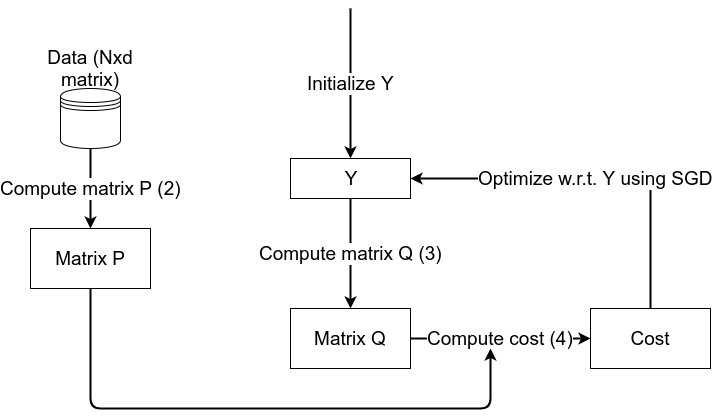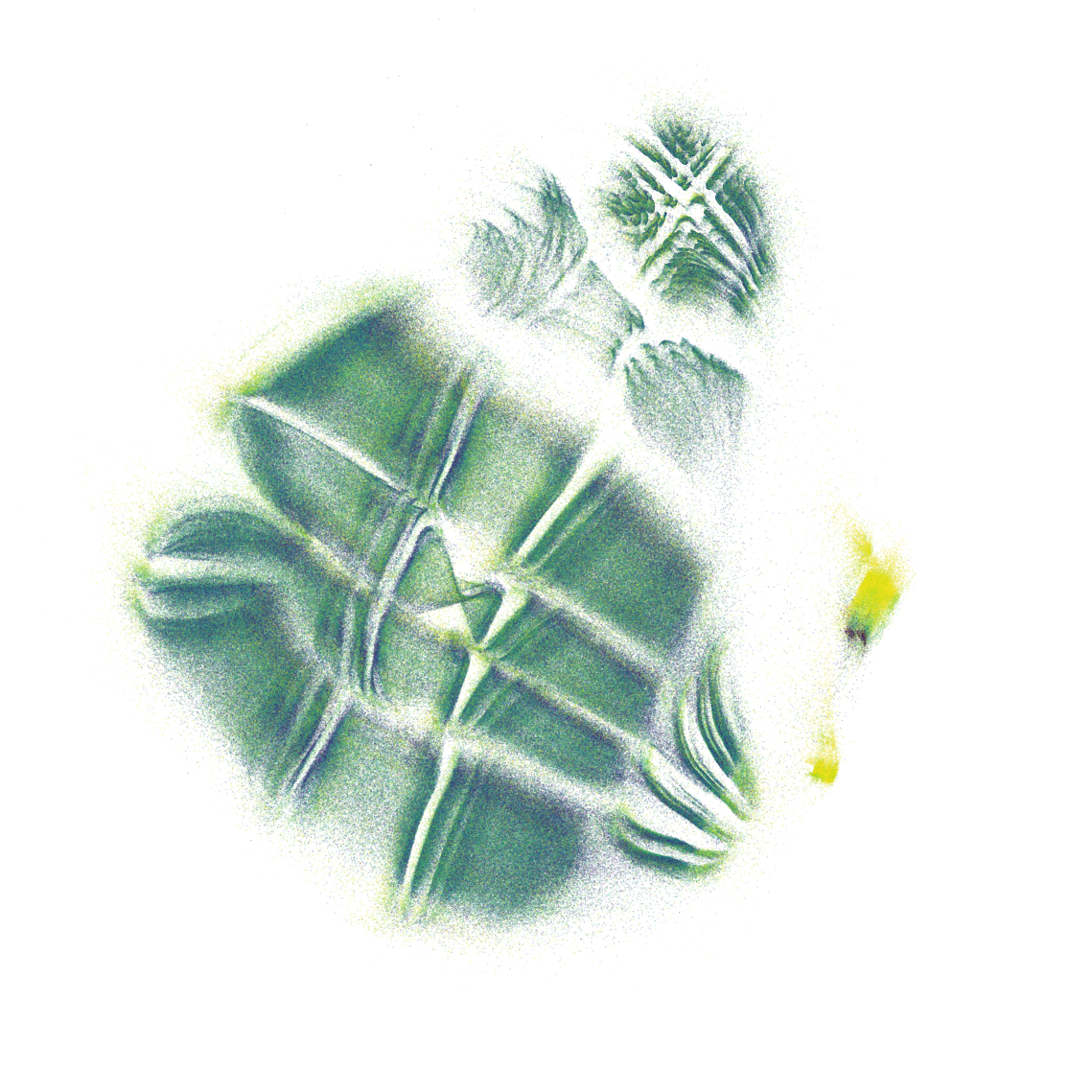# Automatic feature extraction with t-SNE

June 22, 2020

A common problem data scientists face nowadays is dealing with very high-dimensional data (lots of features). Most of the algorithms for classification and prediction that work for low-dimensional datasets don’t work as well when you have hundreds of features, a problem commonly referred as the curse of dimensionality. To solve this, we can reduce the amount of dimensions by feature selection or feature extraction, usually handpicking the most relevant features or using Principal Component Analysis (PCA) before feeding the data into our favorite model. Even though PCA is amazing in most scenarios, it still is a linear model, which might not be powerful enough to apply to some datasets.

## t-SNE

t-Distributed Stochastic Neighbor Embedding (t-SNE) [Original paper] is a very popular and state of the art dimensionality reduction technique that is usually used to map high dimensional data to 2 or 3 dimensions in order to visualize it. It does so by computing affinities between points, and trying to maintain these affinities in the new, low-dimensional space. A nice post to learn with examples and code about t-SNE can be found here.

Quoting from the original paper:

The similarity of datapoint x to datapoint y is defined as the conditional probability, p(y|x) , that x would pick y as its neighbor if neighbors were picked in proportion to their probability density under a Gaussian centered at x .

Here I denote X as a matrix containing the dataset, where each row is a sample and Y the matrix containing the representations in low-dimensional space (which we want to find). Similarity between 2 points in high-dimensional space is given by:

The affinity metric we use is a symmetric version of this equation, so that the affinity of A to B is the same as B to A, which is given by:

For the low dimensional space, t-SNE uses a student-t distribution instead, as it has an easier and cheaper to compute gradient, giving us the formula for affinities in low dimensional space (for d dimensions):

So now that we have the affinities for each pair of points, and we want to keep the low-dimensional ones as close as possible to the original ones, we need to have a loss function that measures the distances between those similarities. Since we defined the similarities as probabilities, a common loss function to use between probability distributions is the Kullback-Liebler divergence, defined as

And our goal is to find Y (the low-dimensional embeddings) that minimize this loss function, for which we can use for example Stochastic Gradient Descent (SGD).Figure 1. t-SNE algorithm workflow. The loop only stops when Y doesn’t change much between iterations

Let’s go over the algorithm step by step, illustrated by Figure 1.

1. Compute matrix P from the Data using Equation (1)
2. Initialize Y (the embeddings) randomly
3. Compute matrix Q from current Y using Equation (2)
4. Compute cost from matrix P and Q using Equation (3)
5. Compute the gradient of the cost with respect to Y and update Y
6. Go back to step 3.

After stopping the algorithm, we end up with our Y as the embeddings of the whole dataset.

There’s also a small step we are missing here to solve the problem of computing σ in Equation (1). Usually a perplexity level is provided by the user which allows us to compute this parameter, but I won’t go into detail in this post. You can get a better insight about this parameter in this post which explains very well what it is and how to use it.

There are, however, some drawbacks related to t-SNE:

• It is not parametric, meaning you can’t find the low-dimensional embeddings of new points that weren’t used in the training phase without running the algorithm again
• It is not scalable, meaning you need the whole dataset and matrices P and Q in memory which won’t work when your dataset doesn’t fit in memory

The main reason this work was done was to address these drawbacks.

## Dealing with new points

The t-SNE algorithm finds those embeddings (Y) for all the dataset that was used, but what if we want to find the embedding of a new point after we have trained the model? Well, you can’t with a vanilla t-SNE. In other words, originally t-SNE was not a parametric model, but the author created a model a few years later that bypasses this limitation. (See here)

A way to create a parametric t-SNE is using a feed-forward neural network to map a high-dimensional point (x) to its low-dimensional embedding (y), and optimizing the t-SNE loss function with respect to the neural networks weights. An implementation in Keras can be found here.

Let’s go over the algorithm step by step, illustrated by Figure 2.

1. Compute matrix P from the Data using Equation (1)
2. Feed the data into the neural network to get Y as the output (a.k.a. forward pass)
3. Compute matrix Q from Y using Equation (2)
4. Compute cost from matrix P and Q using Equation (3)
5. Compute the gradient of the cost with respect to neural network’s weights and update them (a.k.a. backward pass)
6. Go back to step 2.

After training, we end up with a neural network that takes as input a high-dimensional point (x) and outputs its embedding (y), which we can use on data that t-SNE has never seen before!

## Dealing with big datasets

A big limitation of t-SNE is the poor scalability of the matrices P and Q with the size of the dataset. If, for example, we have 1 million samples (which occupies some MB of memory) and we want to run t-SNE on it, we’d end up with a matrix with 10¹² entries, which would occupy roughly 1 TB of memory!

There is an approximation called barnes-hut t-SNE (not parametric though) released by the same author. This version computes the (approximated) nearest neighbors for each point and then only computes the pairwise affinities for the nearest neighbors instead of the whole dataset and assumes the rest are zero. You no longer need a N² matrix in memory (if you use a sparse representation), but you still need the whole dataset in memory as the algorithm doesn’t work in batches.

Instead we’ll use a simple way around the problem which feels more natural with neural networks.

What we’ll do is to feed the neural network in batches and to simply compute P and Q (Eq. 2 and 3) only within each batch, that is, we’ll compute the pairwise distances between points that are in the same batch. That reduces the complexity immensely and allows us to run t-SNE on datasets of virtually any size.

## Experiments

To demonstrate the power of this scalable t-SNE, we’ll use a big dataset (> 1 million samples) from kaggle, the NYC taxi dataset.

The goal of this kaggle competition is to predict the trip duration of a taxi, given its pickup location, date, time of day, vendor and number of passengers. We have extracted some features from the data, such as day of week and month.

Let’s run t-SNE (removing the target column) and see what we find out.Figure 4. Embeddings of the dataset after running t-SNE, colored by trip duration, using a logarithmic scale. Lighter means longer trip time.

We can clearly see 3 big clusters in the data, and 2 smaller ones in the right with significantly longer trip duration. What could they be? Let’s color them by different features and see if we can find the structure uncovered by t-SNE.

The smaller, high trip duration clusters all have the similar pickup coordinates or dropoff coordinates, and it turns out to be the international airport! Actually in the middle of the airport clusters there’s a short trip time cluster which corresponds to going from somewhere around the airport to not too far from it. Those other 3 big clusters clearly correspond to a division in number of passengers, one for one and two passengers, one for 3 passengers, and another one for more than 3 passengers. Every other feature is clearly organized in the 2D space. t-SNE did a great job transforming this 8-dimensional space to a 2-dimensional space!

It’s quite interesting visualizing high dimensional data in 2D, and it can be of real value when you have a huge dataset and you have no idea what it might be. However, if you try the scikit-learn implementation of t-SNE with this dataset, it might take a very long while to complete.

After running t-SNE you could use the embeddings as features for a regressor, and get a decent score or outlier detection as seen in this blogpost. Of course it doesn’t make sense to reduce dimensionality in this dataset because it only has 8 features, but when you have thousands of features it might be worthwhile to try t-SNE as a dimensionality reduction technique.

## Conclusion

t-SNE is a very poweful method for data visualization, dimensionality reduction and can even be used for outlier detection. Parameterizing t-SNE gives us extra flexibility and allows it to be combined with other kinds of neural networks. It also allows us to use mini batches which scale to virtually any dataset size.

This was work was done while in an internship at jungle.ai.